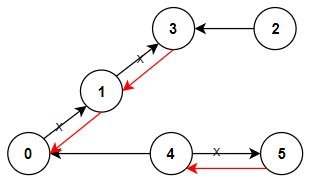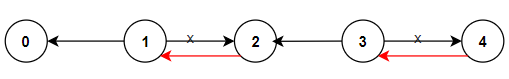Formatted question description: https://leetcode.ca/all/1466.html

# 1466. Reorder Routes to Make All Paths Lead to the City Zero

Medium

## Description

There are n cities numbered from 0 to n-1 and n-1 roads such that there is only one way to travel between two different cities (this network form a tree). Last year, The ministry of transport decided to orient the roads in one direction because they are too narrow.

Roads are represented by connections where connections[i] = [a, b] represents a road from city a to b.

This year, there will be a big event in the capital (city 0), and many people want to travel to this city.

Your task consists of reorienting some roads such that each city can visit the city 0. Return the minimum number of edges changed.

It’s guaranteed that each city can reach the city 0 after reorder.

Example 1:Input: n = 6, connections = [[0,1],[1,3],[2,3],[4,0],[4,5]]

Output: 3

Explanation: Change the direction of edges show in red such that each node can reach the node 0 (capital).

Example 2:Input: n = 5, connections = [[1,0],[1,2],[3,2],[3,4]]

Output: 2

Explanation: Change the direction of edges show in red such that each node can reach the node 0 (capital).

Example 3:

Input: n = 3, connections = [[1,0],[2,0]]

Output: 0

Constraints:

• 2 <= n <= 5 * 10^4
• connections.length == n-1
• connections[i].length == 2
• 0 <= connections[i], connections[i] <= n-1
• connections[i] != connections[i]

## Solution

Since the paths form a tree where city 0 is the root, calculate the cities’ levels using connections, where city 0 has level 0. For each edge, if the source city’s level is less than the destination city’s level, then the edge’s direction needs to be changed. Calculate the number of edges that need to change the direction and return the number.

class Solution {
public int minReorder(int n, int[][] connections) {
Map<Integer, Set<Integer>> edgeMap = new HashMap<Integer, Set<Integer>>();
for (int[] connection : connections) {
int node0 = connection, node1 = connection;
Set<Integer> set0 = edgeMap.getOrDefault(node0, new HashSet<Integer>());
Set<Integer> set1 = edgeMap.getOrDefault(node1, new HashSet<Integer>());
edgeMap.put(node0, set0);
edgeMap.put(node1, set1);
}
Map<Integer, Integer> nodeLevelMap = new HashMap<Integer, Integer>();
nodeLevelMap.put(0, 0);
Queue<Integer> queue = new LinkedList<Integer>();
queue.offer(0);
while (!queue.isEmpty()) {
int node = queue.poll();
int level = nodeLevelMap.get(node);
int nextLevel = level + 1;
Set<Integer> set = edgeMap.getOrDefault(node, new HashSet<Integer>());
for (int nextNode : set) {
if (!nodeLevelMap.containsKey(nextNode)) {
nodeLevelMap.put(nextNode, nextLevel);
queue.offer(nextNode);
}
}
}
int reorderCount = 0;
for (int[] connection : connections) {
int node0 = connection, node1 = connection;
int level0 = nodeLevelMap.get(node0), level1 = nodeLevelMap.get(node1);
if (level0 < level1)
reorderCount++;
}
return reorderCount;
}
}